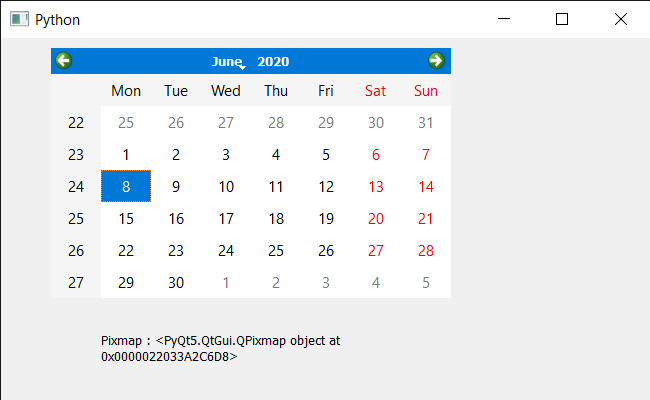Related Articles

# PyQt5 QCalendarWidget – Grabbing Rectangle

• Last Updated : 09 Jun, 2020

In this article we will see how we can grab the rectangle from the QCalendarWidget. Grabbing rectangle means to renders the calendar into a pixmap restricted by the given rectangle. If the calendar has any children, then they are also painted in the appropriate positions.

In order to do this we will use `grab` method with the QCalendarWidget object.

Syntax : calendar.grab(rectangle)

Argument : It takes QRect object as argument

Return : It return QPixmap object

Below is the implementation

 `# importing libraries``from` `PyQt5.QtWidgets ``import` `*` `from` `PyQt5 ``import` `QtCore, QtGui``from` `PyQt5.QtGui ``import` `*` `from` `PyQt5.QtCore ``import` `*` `import` `sys`` ` ` ` `class` `Window(QMainWindow):`` ` `    ``def` `__init__(``self``):``        ``super``().__init__()`` ` `        ``# setting title``        ``self``.setWindowTitle(``"Python "``)`` ` `        ``# setting geometry``        ``self``.setGeometry(``100``, ``100``, ``650``, ``400``)`` ` `        ``# calling method``        ``self``.UiComponents()`` ` `        ``# showing all the widgets``        ``self``.show()`` ` `    ``# method for components``    ``def` `UiComponents(``self``):`` ` `        ``# creating a QCalendarWidget object``        ``self``.calendar ``=` `QCalendarWidget(``self``)`` ` `        ``# setting geometry to the calender``        ``self``.calendar.setGeometry(``50``, ``10``, ``400``, ``250``)`` ` `        ``# setting cursor``        ``self``.calendar.setCursor(Qt.PointingHandCursor)`` ` `        ``# creating label to show the properties``        ``self``.label ``=` `QLabel(``self``)`` ` `        ``# setting geometry to the label``        ``self``.label.setGeometry(``100``, ``280``, ``250``, ``60``)`` ` `        ``# making label multi line``        ``self``.label.setWordWrap(``True``)`` ` `        ``# rectangle``        ``rec ``=` `QRect(``50``, ``10``, ``100``, ``100``)`` ` `        ``# grab``        ``value ``=` `self``.calendar.grab(rec)`` ` `        ``# setting text to the label``        ``self``.label.setText(``"Pixmap : "` `+` `str``(value))`` ` ` ` `# create pyqt5 app``App ``=` `QApplication(sys.argv)`` ` `# create the instance of our Window``window ``=` `Window()`` ` `# start the app``sys.exit(App.``exec``())`

Output :Attention geek! Strengthen your foundations with the Python Programming Foundation Course and learn the basics.

To begin with, your interview preparations Enhance your Data Structures concepts with the Python DS Course. And to begin with your Machine Learning Journey, join the Machine Learning – Basic Level Course

My Personal Notes arrow_drop_up